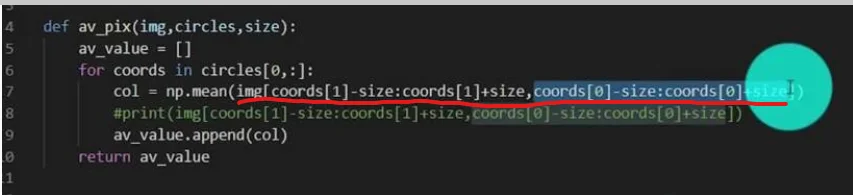09 Aug 2022

Posted on:

07 Aug 2022

0

in line 7
col = np.mean(....)

what is the img value to calculate mean?Instructor
Posted on:

09 Aug 2022

1

Hey Pajjaphon,

If I understand correctly, you are confused about what is happening inside the `mean` function.

It's important to understand what each of the parameters of the `av_pix` function are.
`img` is a numpy ndarray having dimensions (890, 1920). This you can confirm by adding 2 `print`-statements after the definition of `img`, namely:

``````img = cv2.imread('capstone_coins.png', cv2.IMREAD_GRAYSCALE)
img = cv2.GaussianBlur(img, (5, 5), 0)
print(type(img))
print(img.shape)
``````

`circles` is a numpy ndarray of dimensions (1, 8, 3). Therefore, `circles[0, :]` is a numpy ndarray of dimensions (8, 3). Each of the 8 rows represents a coin and each coin is described by 3 numbers - the pixel corresponding to the x-coordinate of the center of the coin, the pixel corresponding to the y-coordinate, and finally the radius of the coin. This you can confirm by adding several `print`-statements after the definition of the `circles` variable:

``````circles = cv2.HoughCircles(img, cv2.HOUGH_GRADIENT, 0.9, 120, param1=50, param2=27, minRadius=60, maxRadius=120)
circles = np.uint16(np.around(circles))
print(type(circles))
print(circles.shape)
print(circles[0, :].shape)
``````

Finally, `size` is an integer given on line 41 when calling the `av_pix` function. Its value is 20.

Let's now go to the definition of the `av_pix` function on line 4. I will add several `print`-functions that will guide us through the process:

``````def av_pix(img, circles, size):
av_value = []

for coords in circles[0, :]:
print(f'coords: {coords}')
col = np.mean(img[coords - size:coords + size, coords - size:coords + size])

print(f'sliced img shape: {img[coords-size:coords+size, coords-size:coords+size].shape}')
print(f'average value: {col}\n')

av_value.append(col)

return av_value
``````

Let's concentrate on line 7 in Giles' code.
The `coords` iterator represents each of the coins. Therefore, `coords` corresponds to the x-coordinate and `coords` corresponds to the y-coordinate. Let's take the first coin, such that
`coords = 1358`
`coords = 254`
`size = 20`
Let's now try and understand what the following slice represents

``````coords - size:coords + size
``````

It corresponds to
`254-20:254+20`
which results in the following slice
`234:274`.
Therefore, we are taking all 40 rows, starting from index 234 all the way to index 273 (note that the first number is included, while the second is not)..

Analogously, the following second slice

``````coords - size:coords + size
``````

corresponds to
`1358-20:1358+20`
which results in
`1338:1378`.
Therefore, we are also taking all 40 columns starting from index 1338 all the way to index 1377.

This has resulted in a 40x40 square matrix whose center is the center of the first coin. The mean of all numbers in the matrix is then calculated and stored in the `col` variable. This value is then appended to the `av_value` list and the procedure is then repeated for the 7 other coins.

Hope this helps! Try to play around with the `print`-functions, make sure you understand the outputs, and you will have no trouble understanding the whole algorithm :)

Kind regards,
365 Hristina